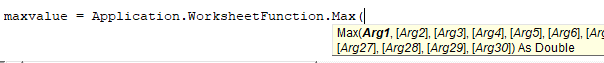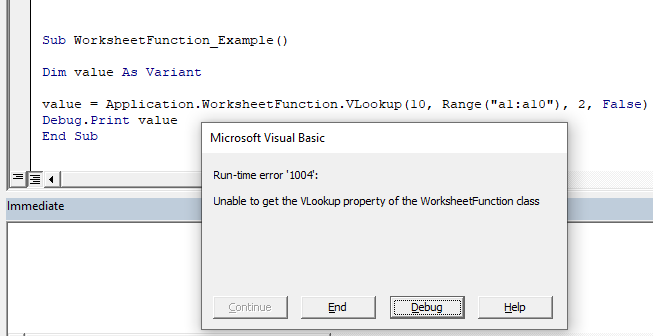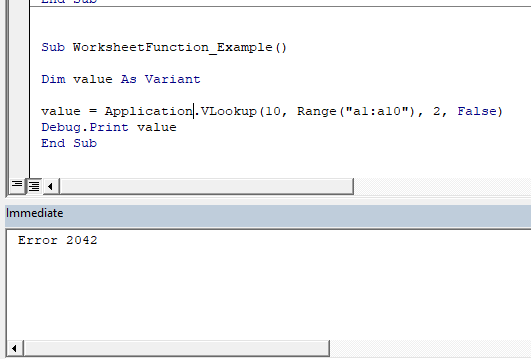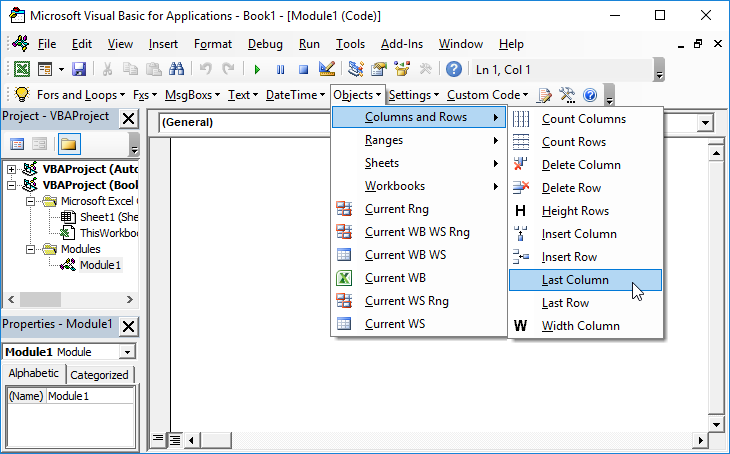# VBA – How to Use Worksheet Functions (and a Full List)

There are many ways to use functions in VBA.  VBA comes loaded with many built-in functions. You can even create your own functions (UDFs). However, you can also utilize many of Excel’s functions in VBA by using Application.WorksheetFunction.

## How to Use Worksheet Functions in VBA

To access an Excel function in VBA add Application.WorksheetFunction in front of the function that you wish to call. In the example below, we’ll call Excel’s Max Function:

``````Dim maxvalue as long
maxvalue = Application.WorksheetFunction.Max(Range("a1").Value, Range("a2").Value)``````

The syntax of the functions are the same, however you will enter the function arguments just like you would any other VBA function.

Notice that the syntax of the Max Function appears when you type (similar to with VBA Functions):### WorksheetFunction Method

WorksheetFunction is a method of Application object.  It allows you access to many (not all) of the standard Excel worksheet functions. Generally, you won’t gain access to any worksheet functions that have a corresponding VBA version.

You can see a list of many of the most common Worksheet Functions below.

## Application.WorksheetFunction vs. Application

There are actually two ways to access these functions:

Application.WorksheetFunction (as seen above):

``maxvalue = Application.WorksheetFunction.Max(Range("a1").Value, Range("a2").Value)``

or you can omit the WorksheetFunction

``maxvalue = Application.Max(Range("a1").Value, Range("a2").Value)``

Unfortunately, omitting the WorksheetFunction will eliminate the Intellisense that displays the syntax (see image above). However, it has one big potential advantage: Error Handling.

If you use Application, and your function generates an error it will return the error value.  If you use the WorksheetFunction method, VBA will throw a run time error.  Of course, you can handle the VBA error, but it’s usually better to avoid the error in the first place.

Let’s look at an example to see the difference:

### Vlookup WorksheetFunction Error Handling

We will attempt to perform a Vlookup that will not result in a match. So the Vlookup function will return an error.

First, we will use the WorksheetFunction method. Notice how VBA throws an error:

Next we omit the WorksheetFunction. Notice how theNext we will omit the WorksheetFunction.  Notice how no error is thrown and instead the ‘value’ function contains the error value from the Vlookup.## VBA Worksheet Functions List

Below you will find a list of most of the common VBA WorksheetFunctions.

FunctionDescription
Logical
ANDChecks whether all conditions are met. TRUE/FALSE
IFIf condition is met, do something, if not, do something else.
IFERRORIf result is an error then do something else.
ORChecks whether any conditions are met. TRUE/FALSE
Lookup & Reference
CHOOSEChooses a value from a list based on it's position number.
HLOOKUPLookup a value in the first row and return a value.
INDEXReturns a value based on it's column and row numbers.
LOOKUPLooks up values either horizontally or vertically.
MATCHSearches for a value in a list and returns its position.
TRANSPOSEFlips the oriention of a range of cells.
VLOOKUPLookup a value in the first column and return a value.
Date & Time
DATEReturns a date from year, month, and day.
DATEVALUEConverts a date stored as text into a valid date
DAYReturns the day as a number (1-31).
DAYS360Returns days between 2 dates in a 360 day year.
EDATEReturns a date, n months away from a start date.
EOMONTHReturns the last day of the month, n months away date.
HOURReturns the hour as a number (0-23).
MINUTEReturns the minute as a number (0-59).
MONTHReturns the month as a number (1-12).
NETWORKDAYSNumber of working days between 2 dates.
NETWORKDAYS.INTLWorking days between 2 dates, custom weekends.
NOWReturns the current date and time.
SECONDReturns the second as a number (0-59)
TIMEReturns the time from a hour, minute, and second.
TIMEVALUEConverts a time stored as text into a valid time.
WEEKDAYReturns the day of the week as a number (1-7).
WEEKNUMReturns the week number in a year (1-52).
WORKDAYThe date n working days from a date.
YEARReturns the year.
YEARFRACReturns the fraction of a year between 2 dates.
Engineering
CONVERTConvert number from one unit to another.
Financial
FVCalculates the future value.
PVCalculates the present value.
NPERCalculates the total number of payment periods.
PMTCalculates the payment amount.
RATECalculates the interest Rate.
NPVCalculates the net present value.
IRRThe internal rate of return for a set of periodic CFs.
XIRRThe internal rate of return for a set of non-periodic CFs.
PRICECalculates the price of a bond.
INTRATEThe interest rate of a fully invested security.
Information
ISERRTest if cell value is an error, ignores #N/A. TRUE/FALSE
ISERRORTest if cell value is an error. TRUE/FALSE
ISEVENTest if cell value is even. TRUE/FALSE
ISLOGICALTest if cell is logical (TRUE or FALSE). TRUE/FALSE
ISNATest if cell value is #N/A. TRUE/FALSE
ISNONTEXTTest if cell is not text (blank cells are not text). TRUE/FALSE
ISNUMBERTest if cell is a number. TRUE/FALSE
ISODDTest if cell value is odd. TRUE/FALSE
ISTEXTTest if cell is text. TRUE/FALSE
TYPEReturns the type of value in a cell.
Math
ABSCalculates the absolute value of a number.
AGGREGATEDefine and perform calculations for a database or a list.
CEILINGRounds a number up, to the nearest specified multiple.
COSReturns the cosine of an angle.
DEGREESConverts radians to degrees.
DSUMSums database records that meet certain criteria.
EVENRounds to the nearest even integer.
EXPCalculates the exponential value for a given number.
FACTReturns the factorial.
FLOORRounds a number down, to the nearest specified multiple.
GCDReturns the greatest common divisor.
INTRounds a number down to the nearest integer.
LCMReturns the least common multiple.
LNReturns the natural logarithm of a number.
LOGReturns the logarithm of a number to a specified base.
LOG10Returns the base-10 logarithm of a number.
MROUNDRounds a number to a specified multiple.
ODDRounds to the nearest odd integer.
PIThe value of PI.
POWERCalculates a number raised to a power.
PRODUCTMultiplies an array of numbers.
QUOTIENTReturns the integer result of division.
RANDBETWEENCalculates a random number between two numbers.
ROUNDRounds a number to a specified number of digits.
ROUNDDOWNRounds a number down (towards zero).
ROUNDUPRounds a number up (away from zero).
SINReturns the sine of an angle.
SUBTOTALReturns a summary statistic for a series of data.
SUMIFSums numbers that meet a criteria.
SUMIFSSums numbers that meet multiple criteria.
SUMPRODUCTMultiplies arrays of numbers and sums the resultant array.
TANReturns the tangent of an angle.
Stats
AVERAGEAverages numbers.
AVERAGEIFAverages numbers that meet a criteria.
AVERAGEIFSAverages numbers that meet multiple criteria.
CORRELCalculates the correlation of two series.
COUNTCounts cells that contain a number.
COUNTACount cells that are non-blank.
COUNTBLANKCounts cells that are blank.
COUNTIFCounts cells that meet a criteria.
COUNTIFSCounts cells that meet multiple criteria.
FORECASTPredict future y-values from linear trend line.
FREQUENCYCounts values that fall within specified ranges.
GROWTHCalculates Y values based on exponential growth.
INTERCEPTCalculates the Y intercept for a best-fit line.
LARGEReturns the kth largest value.
LINESTReturns statistics about a trendline.
MAXReturns the largest number.
MEDIANReturns the median number.
MINReturns the smallest number.
MODEReturns the most common number.
PERCENTILEReturns the kth percentile.
PERCENTILE.INCReturns the kth percentile. Where k is inclusive.
PERCENTILE.EXCReturns the kth percentile. Where k is exclusive.
QUARTILEReturns the specified quartile value.
QUARTILE.INCReturns the specified quartile value. Inclusive.
QUARTILE.EXCReturns the specified quartile value. Exclusive.
RANKRank of a number within a series.
RANK.AVGRank of a number within a series. Averages.
RANK.EQRank of a number within a series. Top Rank.
SLOPECalculates the slope from linear regression.
SMALLReturns the kth smallest value.
STDEVCalculates the standard deviation.
STDEV.PCalculates the SD of an entire population.
STDEV.SCalculates the SD of a sample.
STDEVPCalculates the SD of an entire population
TRENDCalculates Y values based on a trendline.
Text
CLEANRemoves all non-printable characters.
DOLLARConverts a number to text in currency format.
FINDLocates position of text within a cell.Case-sensitive.
LEFTTruncates text a number of characters from the left.
LENCounts number of characters in text.
MIDExtracts text from the middle of a cell.
PROPERConverts text to proper case.
REPLACEReplaces text based on it's location.
REPTRepeats text a number of times.
RIGHTTruncates text a number of characters from the right.
SEARCHLocates position of text within a cell.Not Case-sensitive.
SUBSTITUTEFinds and replaces text. Case-sensitive.
TEXTConverts a value into text with a specific number format.
TRIMRemoves all extra spaces from text.

## VBA Coding Made Easy

Stop searching for VBA code online. Learn more about AutoMacro – A VBA Code Builder that allows beginners to code procedures from scratch with minimal coding knowledge and with many time-saving features for all users!